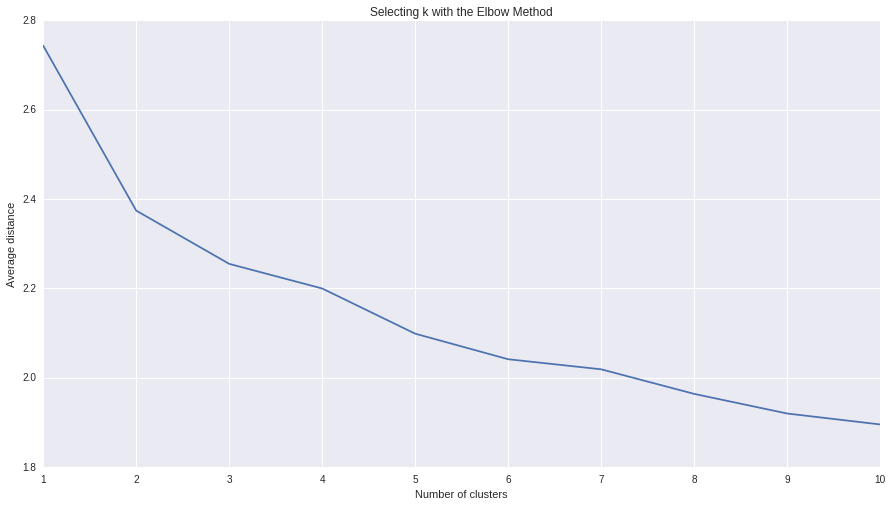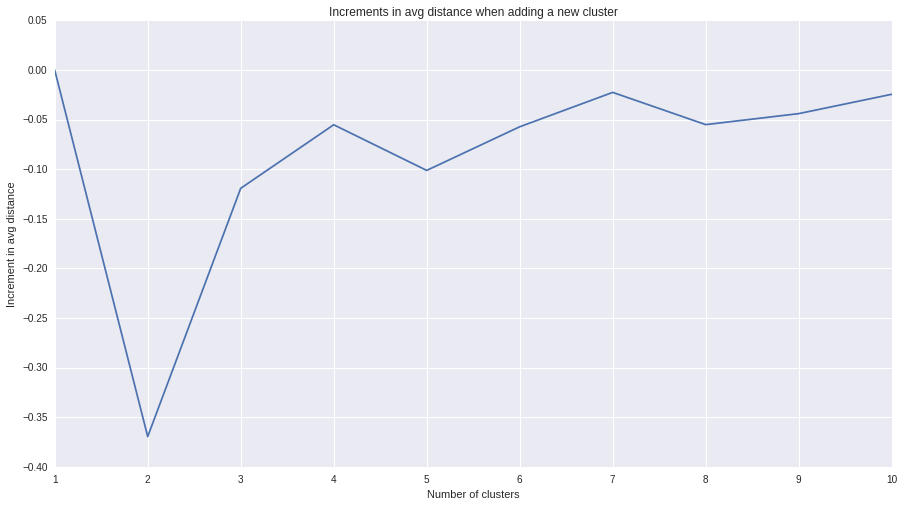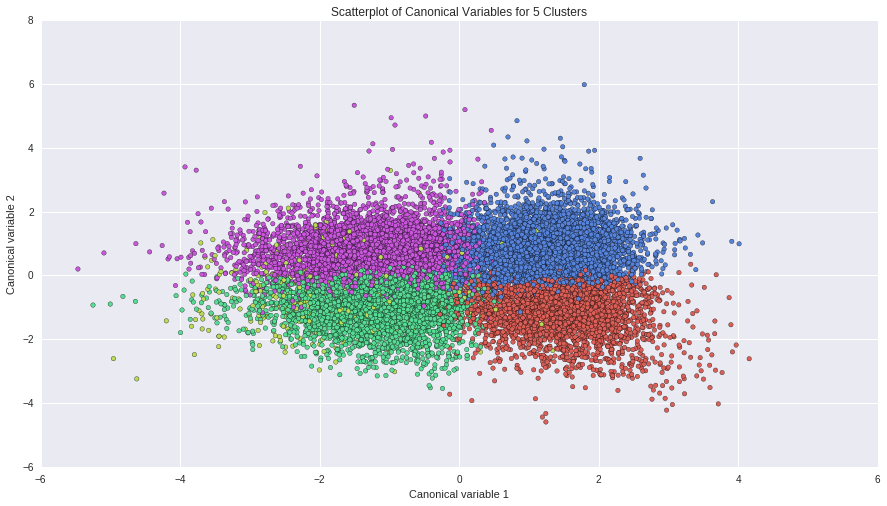# samucoder

Samuel M.H. 's technological blog

Notebook

# Running a k-means Cluster Analysis

Author: Samuel M.H. <samuel.mh@gmail.com> Date: 17-04-2016

## Instructions

This week’s assignment involves running a k-means cluster analysis. Cluster analysis is an unsupervised machine learning method that partitions the observations in a data set into a smaller set of clusters where each observation belongs to only one cluster. The goal of cluster analysis is to group, or cluster, observations into subsets based on their similarity of responses on multiple variables. Clustering variables should be primarily quantitative variables, but binary variables may also be included.

Your assignment is to run a k-means cluster analysis to identify subgroups of observations in your data set that have similar patterns of response on a set of clustering variables.

## What to Submit

Following completion of the steps described above, create a blog entry where you submit syntax used to run a k-means cluster analysis (copied and pasted from your program) along with corresponding output and a brief written summary. Please note that your reviewers should NOT be required to download any files in order to complete the review.

This assignment does NOT require you to run your cluster analysis again on a test data set. You are welcome to do so, but you are only required to run your cluster analysis once on your training data set. If your data set has a relatively small number of observations, you do not need to split into training and test data sets. You can provide your rationale for not splitting your data set in your written summary.

## Dataset

### Variables

• Cluster:
• AGE -> AGE: age in years.
• S1Q24LB -> WEIGHT: weight in pounds.
• S1Q24FT,S1Q24IN-> HEIGHT: height in inches.
• SEX -> MALE: 1 if the subject is a male.
• NUMPERS -> HOUSE_PEOPLE: number of persons in household.
• ETOTLCA2 -> ALCOHOL: average daily volume of ethanol consumed in past year, from all types of alcoholic beverages combined in ounzes.
• S1Q4A -> MARRIAGE: age at first marriage (years).
• S1Q8D -> WORK: age when first worked full time, 30+ hours a week (years).
• S1Q12A -> INCOME: total household income in last 12 months (dolars).
• Statistical test:
• ETHRACE2A -> RACE: race/ethnical group of the subject.
In :
%pylab inline

import numpy as np
import pandas as pd
from scipy.spatial.distance import euclidean
from scipy.stats import chi2_contingency
from sklearn.cluster import KMeans
from sklearn.decomposition import PCA
import statsmodels.formula.api as smf
import statsmodels.stats.multicomp as multi

#Visualization
import matplotlib.pylab as plt
import seaborn as sns

pylab.rcParams['figure.figsize'] = (15, 8)

Populating the interactive namespace from numpy and matplotlib


WARNING: pylab import has clobbered these variables: ['plt']
%matplotlib prevents importing * from pylab and numpy



## Data

In :
# Load data
'ETHRACE2A',
'AGE','S1Q24LB','S1Q24FT','S1Q24IN','SEX','NUMPERS','ETOTLCA2','S1Q4A','S1Q8D','S1Q12A'
])

In :
# Custom dataframe
df = pd.DataFrame()

# Hypothesis to test
df['RACE'] = data['ETHRACE2A'].replace(' ',np.NaN).astype(float)
races = {1:'white',2:'black',3:'indian(US)',4:'asian',5:'latino'}

# Cluster variables
df['AGE'] = data['AGE'].replace(' ',np.NaN).replace('98',np.NaN).astype(float)
df['WEIGHT'] = data['S1Q24LB'].replace(' ',np.NaN).replace('999',np.NaN).astype(float)
df['HEIGHT'] = (
(data['S1Q24FT'].replace(' ',np.NaN).replace(99, numpy.nan)*12) +
data['S1Q24IN'].replace(' ',np.NaN).replace(99, numpy.nan)
).astype(float)
df['MALE'] = data['SEX'].replace(' ',np.NaN).replace('2','0').astype(float)
df['HOUSE_PEOPLE'] = data['NUMPERS'].replace(' ',np.NaN).astype(float)
df['ALCOHOL'] = data['ETOTLCA2'].replace(' ',np.NaN).astype(float)
df['MARRIAGE'] = data['S1Q4A'].replace(' ',np.NaN).replace('99',np.NaN).astype(float)
df['WORK'] = data['S1Q8D'].replace(' ',np.NaN).replace('99',np.NaN).replace('0',np.NaN).astype(float)
df['INCOME'] = data['S1Q12A'].replace(' ',np.NaN).astype(float)

df = df.dropna()

In :
TARGET = 'RACE'
PREDICTORS = list(df.columns)
PREDICTORS.remove(TARGET)

df_predictors = pd.DataFrame()


### Standardize predictors

• 0 Mean
In :
for predictor in PREDICTORS:
pred_data = df[predictor]
df_predictors[predictor] = (df[predictor] - df[predictor].mean()) / df[predictor].std()
df_predictors.describe()

Out:
AGE WEIGHT HEIGHT MALE HOUSE_PEOPLE ALCOHOL MARRIAGE WORK INCOME
count 1.570200e+04 1.570200e+04 1.570200e+04 1.570200e+04 1.570200e+04 1.570200e+04 1.570200e+04 1.570200e+04 1.570200e+04
mean 2.117781e-16 -2.823708e-16 -8.398722e-16 2.104206e-17 9.412361e-17 3.439132e-17 -2.823708e-16 -3.330528e-16 -7.240278e-18
std 1.000000e+00 1.000000e+00 1.000000e+00 1.000000e+00 1.000000e+00 1.000000e+00 1.000000e+00 1.000000e+00 1.000000e+00
min -2.015098e+00 -2.388372e+00 -4.858450e+00 -1.008153e+00 -1.216617e+00 -4.053539e-01 -1.873343e+00 -3.117579e+00 -8.835691e-01
25% -7.548403e-01 -7.321952e-01 -8.146992e-01 -1.008153e+00 -5.127884e-01 -3.916779e-01 -6.963413e-01 -4.496405e-01 -4.718053e-01
50% -8.764499e-02 -1.142190e-01 -5.649585e-02 9.918495e-01 -5.127884e-01 -3.192029e-01 -1.078406e-01 -2.273123e-01 -1.733846e-01
75% 6.536832e-01 5.779145e-01 7.017075e-01 9.918495e-01 8.948696e-01 -1.173722e-02 4.806602e-01 4.396724e-01 1.818782e-01
max 3.618996e+00 6.807115e+00 4.239990e+00 9.918495e-01 7.229331e+00 2.389725e+01 7.738836e+00 1.155608e+01 4.174762e+01

## Model

### K factor selection

In order to choose the optimum value for K, I'm calculating the average distance from a point to its asigned cluster versus for every K from 1 to 10.

In :
K_MAX = 10

In :
#Don't touch!
K_MAX += 1
meandist=[]

for k in range(1,K_MAX):
model=KMeans(n_clusters=k)
clusassign=model.fit_predict(df_predictors)
meandist.append(np.mean([
euclidean(df_predictors.values[i], model.cluster_centers_[cluster])
for i,cluster in enumerate(clusassign)
]))

In :
plt.plot(range(1,K_MAX), meandist)
plt.xlabel('Number of clusters')
plt.ylabel('Average distance')
plt.title('Selecting k with the Elbow Method')

Out:
<matplotlib.text.Text at 0x7f4f5dccb810>In the plot, it is possible to see than adding a new cluster when we have 5 clusters do not decrease much the average distance.

In :
increments = +[ meandist[x]-meandist[x-1] for x in range(1,K_MAX-1)]
plt.plot(range(1,K_MAX), increments)
plt.xlabel('Number of clusters')
plt.ylabel('Increment in avg distance')
plt.title('Increments in avg distance when adding a new cluster')

Out:
<matplotlib.text.Text at 0x7f4f5dbe4c50>This plot represents the increment (or decrement) of the average intra-cluster distance when adding a new cluster. It is possible to see the increment stabilizes from cluster 5 with a peak on K=7 and then decreases logarithmically. So, I say the optimal number of clusters is 5.

In :
K=5


### 5-cluster representation with PCA

In :
#Color palette
colors = sns.color_palette("hls", K)

# Interpret 5 cluster solution
model5=KMeans(n_clusters=K)
model5.fit_predict(df_predictors)

# plot clusters
pca_2 = PCA(2)
plot_columns = pca_2.fit_transform(df_predictors)
plt.scatter(x=plot_columns[:,0], y=plot_columns[:,1], c=[colors[c]for c in model5.labels_])
plt.xlabel('Canonical variable 1')
plt.ylabel('Canonical variable 2')
plt.title('Scatterplot of Canonical Variables for {0} Clusters'.format(K))
plt.show()In :
print('PCA\n -Number of components: {0}\n -Variance explained: {1}'.format(pca_2.n_components_,pca_2.explained_variance_ratio_.sum()))

PCA
-Number of components: 2
-Variance explained: 0.410488357793



The 2-axis plot, coloured with 5 colors (same as the number of clusters), shows 4 clearly distinguishable clusters. This PCA model only captures a 41% of the variance, so I cannot say if the fifth cluster is not easily seen due to the visualization or its contribution to the K-means model.

To test this hypothesis, I will run a Chi-Square test of independence.

In :
df_chi = pd.DataFrame()
df_chi[TARGET] = df[TARGET]
df_chi['CLUSTER'] = model5.labels_

In :
#Contingency table, observations
ct1 = pd.crosstab(df_chi['CLUSTER'],df_chi['RACE'])
print ct1

RACE        1    2   3    4    5
CLUSTER
0        2276  425  50   60  344
1         217   67  11    6   49
2        2552  480  65   52  484
3        2855  567  73  117  961
4        2353  463  56  136  983


In :
#Percentages
colsum = ct1.sum(axis=0)
colpct = ct1/colsum
print (colpct)

RACE            1         2         3         4         5
CLUSTER
0        0.221984  0.212288  0.196078  0.161725  0.121943
1        0.021165  0.033467  0.043137  0.016173  0.017370
2        0.248903  0.239760  0.254902  0.140162  0.171570
3        0.278455  0.283217  0.286275  0.315364  0.340659
4        0.229494  0.231269  0.219608  0.366577  0.348458


In :
# chi-square test
cs1 = chi2_contingency(ct1)
print("X² Value = {0}".format(cs1))
print("p-value = {0}".format(cs1))

X² Value = 384.230240286
p-value = 7.31131755895e-72



The $\chi^2$ test of indepence gives a p-value lesser than 0.05, so the race and the clusster assignation are significantly associated.

### Post hoc test - Bonferroni Adjustment

• Number of categories: 5
• Number of comparisons: $\binom{5}{2} = 10$
• Adjusted p-value: $\frac{p-value}{number of comparisons} = \frac{0.05}{10} = 0.005$
In :
from itertools import combinations
comparison_pairs = list(combinations(races.keys(),2))

for (v1,v2) in comparison_pairs:
print("PAIR: {0}-{1}".format(races[v1],races[v2]))
df2 = df_chi[(df_chi['RACE']==v1) | (df_chi['RACE']==v2)]
ct2 = pd.crosstab(df2['CLUSTER'],df2['RACE'])
cs2 = chi2_contingency(ct2)
print("\t p-value: {0}".format(cs2))
print("\t Reject: {0}".format(cs2<ap_val))

PAIR: white-black
p-value: 0.0147537262383
Reject: False
PAIR: white-indian(US)
p-value: 0.165591902325
Reject: False
PAIR: white-asian
p-value: 5.07966700917e-11
Reject: True
PAIR: white-latino
p-value: 3.09820579923e-68
Reject: True
PAIR: black-indian(US)
p-value: 0.869722513026
Reject: False
PAIR: black-asian
p-value: 4.74267012052e-09
Reject: True
PAIR: black-latino
p-value: 9.03882670447e-35
Reject: True
PAIR: indian(US)-asian
p-value: 2.21147789828e-05
Reject: True
PAIR: indian(US)-latino
p-value: 3.18119315297e-08
Reject: True
PAIR: asian-latino
p-value: 0.136856284896
Reject: False



After the Bonferroni adjustment, these are the two groups statistically different in terms of the 5-groups clustering.

• white-black-indian
• asian-latino

These two superclusters could indicate wether the subject have roots in the country (first group) or they is an inmigrant or a first/secod generation citizen. It will be nice to know wich clusters are associated with the race superclusters

The race seems to be correlated with the output of the clustering process. It is not possible to say the race of a subject knowing their 9 features (AGE, WEIGHT, HEIGHT, MALE, HOUSE_PEOPLE, ALCOHOL, MARRIAGE, WORK and INCOME) but it is possible to assign them to a group.

TODO: know the meaning of the group.

#### 1 comment:

1.very nice analysis, you've gone about it in a easy to understand manner.